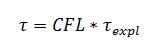### Article Index

To solve a numerical task, it is necessary to discretize space and time. A time scale allows to find intermediate solutions based on the initial and boundary conditions. Gradually coming through the intermediate solutions, we get the final one, which we can use to achieve the goals: choosing the right paint for the rocket skin, calculating the flow rate of your favorite ketchup, or getting the optimal washing temperature for a cat.

The intervals at which software calculates a solution are commonly referred to as the time step. It can be constant or calculated for each iteration based on a certain criteria. It should be noted that the time step is different for each specific software and task.

How to specify it correctly - we will describe in this article.

### Time step in FlowVision

FlowVision allows to set time step as:

• Constant – differential equations will be solved through the equal time intervals during the simulation;
• CFL number – the Courant-Friedrichs-Levy condition (CFL condition) is used. It controls the time step based on the current simulation characteristics.

#### constant time step

Everything is quite boring about constant time step - the timeline is splitted for the required interval (usually less than 0.1 of the characteristic time) and all you have to do - just wait until either the solution converges or the specified computation time ends.

The most common tasks that are solved with constant time step:

• Flow in a tube. The characteristic time for this task is transit time;
• External flow around the body. Characteristic time - the ratio of the body size to the incoming flow velocity;
• Circulating flow. The characteristic time is the time for which a flow particle makes a revolution in the computational domain.

#### CFL number

The CFL criteria are a little more complicated. The criterion itself is a necessary condition for the stability of an explicit numerical solution of partial differential equations. That is, the time step should be less than a certain value, otherwise the simulation results will be non-physical.

To make it clear - FlowVision itself calculates the time step with which it will be comfortable to make calculations based on the already obtained solution. And the user only scales this value.

The step that FlowVision calculates by itself is called the explicit time step (τexpl). The scaling factor is the CFL number. The final time step (τ) is calculated by the formula:If you open Solver tab > Time step, you may see that there are 4 criteria:

• Convective CFL;
• Surface CFL;
• Diffusive CFL;
• Slide CFL.

Each criterion has its' own explicit step, which is calculated by FlowVision:

• Convective explicit step τexpl,conv
• Surface explicit step τexpl,surf
• Diffusive explicit step τexpl,diff
• Slide explicit step τexpl,slide

The limiter for the above steps is

• Gravitational step  τgr .

Maybe, you have a lot of questions already: